Courses

# Test: Linear Algebra - 1

## 20 Questions MCQ Test Topic-wise Tests & Solved Examples for IIT JAM Mathematics | Test: Linear Algebra - 1

Description
This mock test of Test: Linear Algebra - 1 for Mathematics helps you for every Mathematics entrance exam. This contains 20 Multiple Choice Questions for Mathematics Test: Linear Algebra - 1 (mcq) to study with solutions a complete question bank. The solved questions answers in this Test: Linear Algebra - 1 quiz give you a good mix of easy questions and tough questions. Mathematics students definitely take this Test: Linear Algebra - 1 exercise for a better result in the exam. You can find other Test: Linear Algebra - 1 extra questions, long questions & short questions for Mathematics on EduRev as well by searching above.
QUESTION: 1

### Define T(v) = Av, where A =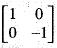. Then T(v):

Solution:

We are given that
T(v) = Av
where A =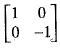We need to define T(v)
Let v =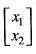,  then T(v) = Av =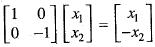Which reflects v about the x2-axis.

QUESTION: 2

### If the linear transformation T(v) = Av rotates the vectors (-1, 0) and (0,1), x radians clockwise, then:

Solution:

We are given that the linear transformation T(v) = Av rotates the vectors (-1,0) and (0,1), π radians clockwise.
We need to find the matrix A.
We know that if a vector (a, b) is rotated through an angle a under T. Then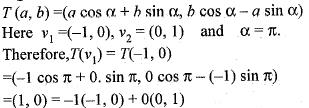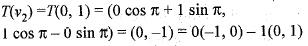Therefore, the matrix of T is
A =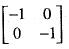QUESTION: 3

### If the linear transformation T(v) = Av rotates the vectors v1 = (-1, 0) and v2 = (0, 1) clockwise π radians, the resulting vectors are:

Solution:

We are give that the linear transformation T(v) = Av rotates the vectors v1 = (-1,0) and v2 = (0,1) clockwise π radians. We need to find the image of v= (-1, 0) and v2 = (0,1) under T
We know that if a vector (a, b) is rotated through an angle α under linear transformation T, then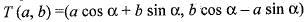Here v1 = (-1, 0) and a = π
Therefore,v2 = (0,1)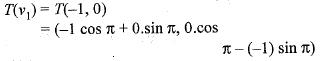= (1,0)
and T(v2) = T(0, 1)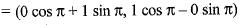= (0,-1)

QUESTION: 4

If the linear transformation T(v) = Av rotates the vectors v1 = (-1, 0) and v2 = (0, 1) clockwise π/2 radians, the resulting vectors are:

Solution:

We are given that the linear transformation T(v) = Av rotates the vector v1 = (-1, 0) and v2 = (0,1) clockwise π/2radians.
We know that if a vector (a, b) is rotated through an angle a clockwise direction under linear transformation T.Then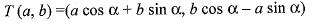Here, v= (-1 , 0) and a = π/2 radians
Therefore, T(v1) = T(-1, 0)
=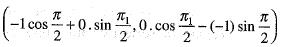= (0,1)
Therefore, T(-1, 0) = (0,1)
Similarly, for v2 = (0, 1) and α = π/2,
and T(v2) = T(0 ,1)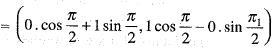= (1,0)
Therefore, T(0,1) = (1 ,0 ).

QUESTION: 5

If the linear transformation T(v) = Av rotates the vectors (-1, 0) and (0, 1) clockwise π/2 radians then:

Solution:

We are given that the linear transformation T(v) = Av rotates the vectors (-1, 0) and (0, 1) clockwise π/2 radians. We need to find the matrix A.
We know that if a vector (a, b) is rotated through an angle a clockwise under T. Then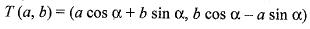Here, (a, b) = (-1, 0)  and a = π/2
Therefore, T (-1, 0)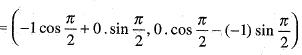= (0, 1) = 0(-1, 0) + 1(0, 1)
and (a, b) = (0,1), α = π/2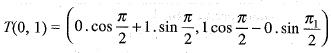= (1, 0) = - 1(-1, 0) + 0(0, 1)
Therefore, the matrix of T is A =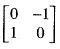QUESTION: 6

If A =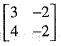satisfies the matrix equation A2 - kA + 2I = 0, then what is the value of k?

Solution:

We need to find the value o f k where the matrix
A =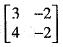satisfies the equations A2 - kA + 2I = 0.
But A =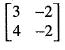So,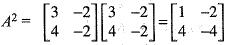Putting these values in the equation
A2 - kA + 2I= 0, we get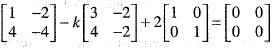or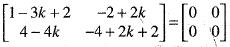or 1 - 3k + 2 = 0 => k = 1

QUESTION: 7

Under which one of the following condition does the system of equations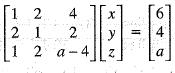have a unique solution?

Solution:

We are given that the system of equationsReduce this system of equations to echelon form, using the operations "R2 ----> + R2 - 2R1" and R3 ---> R3 - R1.
These operations yields: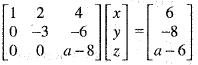Also we have, this system has a unique solution so a - 8 ≠ 0
or a ≠ 8

QUESTION: 8

What is the determinant of the following matrix?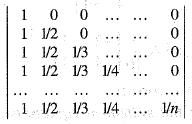Solution:

We need to find the determinant of the given matrix,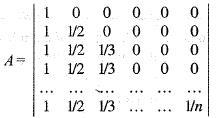determinant of A =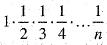or det (A) =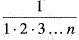det (A) = 1/n!

QUESTION: 9

If the system of equations
x - 2y - 3z = 1, (p + 2)z = 3, (2p + 1)y + z = 2 is inconsistent, then what is the value of P?

Solution:

We are given that the system of equations,
x - 2y - 3z = l, (p + 2)z =3, (2p + l) y + z = 2 is inconsistent. Then we need to find the value of p.
The augmented matrix of this system of equations is,The solution of this matrix is inconsistent if
p + 2 = 0
or p = -2

QUESTION: 10

If x, y, z are in AP with common difference d and the rank of the matrix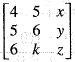is 2, then the value of d and k are

Solution:

We are given that x, y, z are in A.P. with common difference d i. e.,
or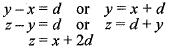and the rank of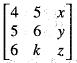is z.
We need to find the values of d and k. The given matrix can be written as,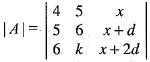Applying R2 -> R2, R1 and R--> R-R2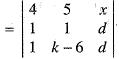Applying, R3 ---> R3 - R2,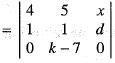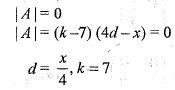QUESTION: 11

The linear transformation T(x, y) =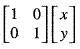can be written as:

Solution:

We have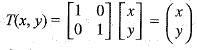or equivalently
T(x,y) = (x,y)

QUESTION: 12

The linear transformation T(x, y) = (x + 2y, x - 2y), can be written as a matrix transformation T(x, y)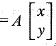where:

Solution:

We are given that a linear transformation defined by
T(x, y) = (x + 2y, x - 2y)
Let B ={(1, 0), (0, 1)} be a standard basis then
T(1,0) = (1,1) = 1 (1 ,0)+ 1(0,1)
T(0, 1) = (2 ,-2) = 2(1,0) - 2(0 ,1)
Therefore, the matrix of T with respect to the basis B is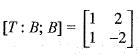QUESTION: 13

Is the transformation T(x, y, z) = (x, y, 0) linear?

Solution:

We are given that a transformation defined by
T(x,y,z) = (x,y, 0)
Let α and β be scalars and (x1, y1, z1) and (x2, y2, z2) be two vectors. Then
T[α(x1, y1, z1) + β(x1, y2, z2)]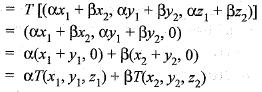or equivalently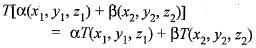Hence, T is linear.

QUESTION: 14

What is the range of T(v) = Av where A =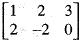?

Solution:

We are given that a linear transformation T defined by
T(v) = Av
where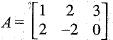Let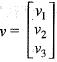Then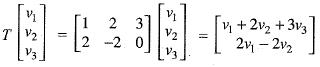Let v1, v2, v3 ∈ ker T. Then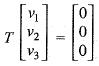Using the definition of T, we get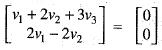or equivalently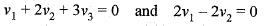or equivalently
v1 = v2 = v3 = - v1
Therefore,ker T=  {(v1, v1, - v1): v ∈ R}.
Therefore, dim ker T= 1
Using Rank-Nullity theorem|
Rank T= 3 - dim ker T
= 3 - 1 = 2
Therefore, the range of T is a plane in R3

QUESTION: 15

Suppose T1: V ---> U and T2 : U —> W be two linear transformation, Then:

Solution:
QUESTION: 16

Which one of the following is true?

Solution:

We need to find the dim Horn (R3, R4).
Since dim R3 = 3 and dim R4 = 4
Therefore,dim Horn (R3, R4)
= 3 x 4 = 12
Let U and V be n and m dimensional vector space.
Then dim Hom (U, V) = nm

QUESTION: 17

If C is a non-singular matrix and B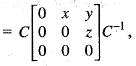then

Solution:

We are given that C is a non-singular matrix and
B =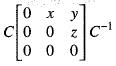or B is similar to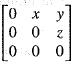The eigen values of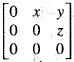are all zeroes.

Hence, Bk = 0
For       k = 3
B3 = 0

QUESTION: 18

If X =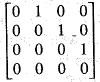,  the rank of XTX, where XT denotes the transpose of X, is

Solution:

We are given that the matrix
X=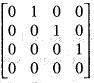We need to find the rank of XTX. The transpose of X,
XT=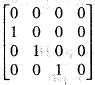Now,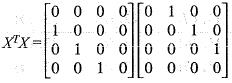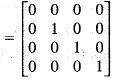Rank (XTX) = 3

QUESTION: 19

The system of equation kx +y + z = 1,x + ky + z = k and x + y + kz = k3 does not have a solution, if k is equal to

Solution:

We are given that the system of equation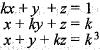does not have a solution. We need to find the value of k. The given linear system of equation can be written as,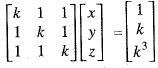This system of equation has no. solution if rank (A) # rank (aug A) the augmented matrix is,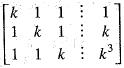This augmented matrix has no. solutions if k = -2.

QUESTION: 20

We are given that the system of equation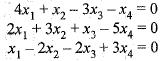has

Solution:

We are given that the system of equation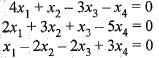It may be written as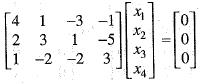Here, the rank of the given coefficient matrix ≤ 3 and number of unknowns are four.
Hence, rank < no. of unknowns this system of equations has infinitely many solutions.